RUSSIAN JOURNAL OF EARTH SCIENCES, VOL. 21, ES3002, doi:10.2205/2021ES000766, 2021

# Numerical algorithms for structural magnetometry inverse problem solving

P. S. Martyshko, N. V. Fedorova, A. L. Rublev

Institute of Geophysics UB RAS, Ekaterinburg, Russia

### Abstract

New methods for studying the anomalous magnetic field of the lithosphere and modeling the sources of anomalies in different layers of the Earth's crust are given in this article. The interpretation consists of three stages: separation of anomalies from the sources in different layers of the Earth's crust, data conversion to the magnetic pole and solving the inverse problem of finding the surface of sources. To isolate anomalies from different layers of the earth's crust, we used a technique based on the subsequent continuation of magnetic data up and down. Calculation of the vertical component of the anomalous magnetic field by its absolute value and conversion to the pole is based on the approximation of the anomalies of the magnetic induction modulus through a set of singular sources – magnetized rods. To solve the nonlinear inverse problem and calculate the boundaries separating layers with different magnetization, the method of local corrections was applied. The new computer technology was created using parallel computing in the multiprocessor computer systems. To demonstrate the developed methods, we chose the region of Western Urals 300 km $\times\: 230$ km, where the large magnetic anomalies are located. Anomalies from various layers of the Earth's crust were identified and the models of the sources of magnetic anomalies in the granite layer and the surface of the basaltic layer were constructed. The study revealed basic-ultramafic massifs under a thick sedimentary cover, as well as extended deep belts and large elevations of the basalt layer of the Earth's crust. The results clarify the lithosphere structure of the Western Urals and can be used for geodynamic reconstructions.

### 1. Introduction

Magnetic anomalies provide important information about the structure and the history of the Earth's lithosphere. Study and analysis of the anomalous field structure have practical value for geological mapping, geological survey, and prospecting for mineral resources, and in the sense of revealing the peculiarities of the tectonic structure of the lithosphere and building geomagnetic models of the Earth's crust.

Magnetic surveys using satellites have allowed the pattern of the magnetic field distribution to be acquired and the centennial variations of the field to be specified over the whole globe, without "white spots". At present, a knowledge about the spatial-temporal peculiarities of the terrestrial magnetic field has been significantly broadened, and the methods of spherical harmonic analysis have been well developed. This has allowed scientists to elaborate the international models of the main magnetic fields (International Geomagnetic Reference Field or briefly IGRF) for every 5-year interval using the unified technique [IAGA Division, 1995].

In the framework of the Federal Special-Purpose Program "Development of prognostic-geophysical maps for the principal mineralgenic zones of Russia", the territory of the Russian Federation has been mapped with digital maps in the past decade. The information basis for the maps were the data of areal airborne and land-based magnetic surveys of large, middle, and small scales made. The geophysical data were processed using modern computer methods. In order to unify the survey data and unite them, observations on the reference airborne magnetic profiles were made and the Uralian mapping network was organized. The correction values of centennial variations in the geomagnetic field were calculated based on the IGRF [IAGA Division, 1995; Olsen, 2002]. Resulting from these works, a digital model of the map for the anomalous geomagnetic field $\Delta Ta$ in the Uralian Region and its vicinity (East European Plain and West Siberia) was created [Chursin et al., 2008]. We used a set of this data to model the sources of magnetic anomalies in the layers of the Earth's crust.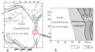Figure 1

We have developed new methods and applied an efficient computer technology to study the structure of the anomalous magnetic field and to build the sources of anomalies in different layers of the Earth's crust. In the study of large anomalies, it is necessary to process big data, therefore, in our new computer technology we used parallel computing on multiprocessor computer systems. The results of using this method are demonstrated for the Western Ural region, covering the Eastern part of the ancient East-European platform and the Western part of the Ural orogeny 300 km $\times\: 230$ km (Figure 1).

The paper is organized as follows: we present mathematical algorithms for separating magnetic field sources in depth, for the approximating of anomalous magnetic field by array of magnetized rods and for 3D magnetic data inversion. Then all algorithms are applied to the Western Urals (Russia) to build the sources of anomalies in different layers of the Earth's crust (to extract the effect of the interfaces between layers and find its topography).

### 2. Methods

#### 2.1. Method of Separation of Anomalies From Sources in the Earth's Crust

To divide the long- and short-wave components of the amplitude spectrum of anomalies, geophysicists utilize numerical methods of field simulation at various altitudes [Martyshko and Prutkin, 2003; Martyshko et al., 2014].

The anomalous magnetic field has an integral character and contains components from all the sources located in the upper lithosphere. In order to extract the anomaly from the sources in different layers of the Earth's crust, a technique based on subsequent upward and downward magnetic data continuation was used [Martyshko et al., 2014]. There are two problems to be solved. Firstly, we continue the observed data upward to the height $H$ to diminish the influence of the sources in the near-surface layer (up to a depth $H$). Then, we continue the obtained function downward to the depth $H$, i.e. to the distance $2H$ in downward direction.

Since the problem of downward continuation is a linear ill-posed inverse problem, therefore we must use some regularization.

Finally, the field was continued upwards again to the Earth's surface. So, we receive the magnetic field of the sources that are located below the depth $H$. Subtracting of this field from the observed one gives the field of the sources that are located within the layer (from Earth's surface to the depth $H$). By repeating this procedure in different heights and depths, we could separate the fields generated by the layers between the corresponding depths.

Let $\{x, y, z\}$ are the rectangular Cartesian coordinates with axis $Z$ pointing downwards and plane $XOY$ coinciding with the surface of the observations and $\Delta T_a = U$. Recalculation of the magnetic field $U(x, y, z)|_{z=0}$ measured on the Earth's surface area upward to the level $z = - H$ is made by the Poisson's formula:

\begin{eqnarray*} \bar{U}(x,y,-H) = \end{eqnarray*} \begin{eqnarray*} \frac{1}{2\pi} \int_{-\infty}^{+\infty} \int_{-\infty}^{+\infty} \frac{-H}{[(x-x')^{2} + (y-y')^{2} + H^{2} ]^{3/2}} \times \end{eqnarray*}

\begin{equation} \tag*{(1)} U(x',y',0) dx' dy'. \end{equation}

Recalculation of the magnetic field $\bar{U}(x,y,-H)$ to the depth $H$ is implemented by the first type of Fredholm equation

\begin{eqnarray*} \frac{1}{2\pi} \int_{-\infty}^{+\infty} \int_{-\infty}^{+\infty} \times \end{eqnarray*} \begin{eqnarray*} \frac{2H}{\left[(x-x')^{2} +(y-y')^{2} +4H^{2} \right]^{3/2}} \times \end{eqnarray*}

\begin{equation} \tag*{(2)} U(x',y',H)dx'dy'=\bar{U}(x,y,-H), \end{equation}

where $U(x',y',H)$ are field values at the depth $H$ to be determined.

Solving the integral equation of the first kind (2) is an ill-posed problem, which requires application of the regularization methods. The operator of equation (2) is positive definite and self-adjoint; therefore we can apply the scheme of Lavrentiev .

After the grid discretization of equation (2) and approximation of the integral operator by the quadrature formulas, the problem is reduced to solving the system of linear algebraic equations (SLAEs) with the symmetric matrix $K$. In the regularized form, the system is

\begin{equation} \tag*{(3)} (K + \alpha I)U = \bar{U}, \end{equation}

where $U$ is the field values on the depth $H$, $I$ is the identity matrix and $\alpha$ is the regularization parameter.

For solving the SLAEs (3) the iteratively regularized simple iteration method was used

\begin{equation} \tag*{(4)} u^{k+1} = u^{k} -\frac{1}{\lambda_{\max}} \left[\left(K + \alpha I \right) u^{k} - \bar{U}\right], \end{equation}

where $\lambda_{\max}$ is the maximal eigenvalue of matrix $K + \alpha I$ (symmetric case), $k$ is the number of iteration.

The stopping criterion in the iterative processes (4) is the fulfillment of the condition

\begin{eqnarray*} \frac{\left\| Ku^{k} -\bar{U}\right\| }{\left\| \bar{U}\right\| } <\varepsilon \end{eqnarray*}

with a sufficiently small $\varepsilon$.

Parallel algorithms. At the stage of upward recalculation of the magnetic field during calculation of integrated operator (1), the parallel algorithm of the matrix to vector multiplying is used. Paralleling of the gradient type iteration methods is based on splitting the $K$ matrix by vertical bands into $m$ blocks, and the solution vectors $u$ and the vectors $U$ from the right part of the system of algebraic equations into $m$ segments, in such a way that $n = m \times L$, where $n$ is the dimension of the equation system and $m$ is the number of processors.

At any iteration, each of $m$ cores (processors) computes its part of the solution vector. If the $K$ matrix is multiplied by the $u$ vector, each one of $m$ cores (processors) multiplies only the respective part of lines from the $K$ matrix to the $u$ vector. The host processor performs data transfer and also computes its part of the solution vector.

The parallel algorithms of the height transforms based on the gradient type iterative methods have been designed and numerically implemented on Uran supercomputer at the Institute of Mathematics and Mechanics of the Ural Branch of the Russian Academy of Sciences [Martyshko et al., 2014]. The Uran supercomputer is a cluster of multiprocessor systems, each computing unit of which is based on two 4-core Intel Quad-Core Xeon processors with a speed of 3 GHz and a 16/32 Gb cache. Overall, 1664 computing cores and 3584 Gb cache memory are available to the user.

We note that for solving the problem by the double-precision iterative methods on the Uran supercomputer, significant memory is required for storing matrix $K$. For the $300 \times 300$ grid, the number of the elements of matrix $K$ takes 60.3 Gb, while the code-run system on the Uran cluster normally allocates at most 2 Gb for each separate process of the MPI program. Therefore, matrix $K$ is preliminarily divided by the vertical bands into blocks and is stored in the memory of each processor by parts. Thus, considering the memory constraints, at least 50 Uran processors are required to solve our problem.

The computational experiments show that for large data volumes, paralleling the algorithms significantly reduces the time of the computations [Martyshko et al., 2014].

#### 2.2. The Approximation of Anomalous Magnetic Field by Array of Magnetized Rods

The magnetic surveys are mainly carrying out on the equipment that measured the absolute values of the geomagnetic field $T$. So the anomalous magnetic field of the lithosphere is often represented by $\Delta Ta$. Since, $\Delta Ta$ is not a harmonic function, for correct application of methods for magnetic inverse problems solving it is necessary to determine the vertical component $Z$. The calculation method for the vertical component of an anomalous magnetic field based on its absolute value was developed. Conversion is based on the approximation of magnetic induction module anomalies through a set of singular sources and the subsequent calculation for the vertical component of the field with the chosen distribution. In a paper [Mayer et al., 1985], it is proposed to use a set of rods that are uniformly magnetized along a geomagnetic field. Each rod is determined by seven parameters: six coordinates of two edge and magnetization of the source. Magnetic anomalies from such sources are expressed through algebraic dependencies and are calculated very quickly. It was shown that a small number of such sources can well approximate complicated magnetic anomalies [Mayer et al., 1985].

The approximation reduces to solving the following nonlinear programming problem: minimize the functional, which is the difference between the measured data and the field of model sources:

\begin{equation} \tag*{(5)} F(N,p) = \sum_{i=1}^{M} (\Delta Ta_{i} - f(N,p,x_{i},y_{i},z_{i} ))^{2}, \end{equation}

where $\Delta Ta_{i}$ is the field values specified at the points $(x_i, y_i, z_i)$ on observation surface (may be in irregular grid); $N$ is the number of objects involved in the procedure; $P$ is the vector of rod parameters with dimension $7 \times N$; $f$ is the function that calculates the total field value of $N$ rods, parameters of which are determined by the vector $P$ at the points $(x_i, y_i, z_i)$.

Model rods are small parametric of source class, it increases stability of the inverse problem solution. To minimize the functional (5), various nonlinear programming methods were used and the Polack-Ribier method was selected, which converges faster than the others [Byzov et al., 2017]. The algorithm was implemented using the parallel computing technology on the NVIDIA GPU (GeForce GTX 780).

The method allows high-precision approximation of complex magnetic anomalies. Using the solution of the direct problem for the found model rods, it is easy to calculate the values of $Z$ for the vertical direction of the magnetization vector. For anomalies, the intensity of which varies from 1000 to 3000 nT, the error of the obtained values is estimated as $\pm 20$ nT. Note the conversion to the magnetic pole can significantly reduce the computational process for solving the inverse problem to find the geometry of the volume sources.

#### 2.3. Method to Solve the Inverse Problem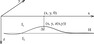Figure 2

Let $\{x, y, z\}$ are the rectangular Cartesian coordinates with axis $Z$ pointing downwards and plane $XOY$ coinciding with the surface of the observations. We consider the two-layer medium in 3D space. The model consists of two layers of a constant magnetization. Surface separating the upper and lower layers at a sufficient distance from the center of area goes to the asymptote (Figure 2).

We calculated the vertical component of the magnetic induction $Z$ of the contact surface, separated the layers with vertical magnetization, $I_1$ and $I_2$, at the point $(x, y, 0)$ on the ground surface by the formula:

\begin{eqnarray*} Z(x,y,0) = \Delta I\int_{-\infty}^{+\infty}\int_{-\infty}^{+\infty} \times \end{eqnarray*} \begin{eqnarray*} \left( \frac{z(x,y)}{\left[(x-x')^{2} + (y-y')^{2} + z^{2} (x,y)\right]^{3/2}} - \right. \end{eqnarray*}

\begin{equation} \tag*{(6)} \left. \frac{H}{\left[(x-x')^{2} + (y-y')^{2} + H^{2} \right]^{3/2}} \right)dxdy, \end{equation}

where $z =z(x,y)$ is the equation of the surface separating the upper and lower layers, the $\Delta I$ is the magnetization jump at the boundary layers, $H$ is the horizontal asymptote.

To solve the equation (6) and find the function $z(x,y)$ we use algorithm based on the modified method of local corrections [Martyshko et al., 2010]. Method of local corrections was proposed for the approximate solution of nonlinear inverse gravimetric problems [Prutkin, 1986]. It is based on the assumption that the variation of the field at a certain point is affected mostly by the variation of the part of the object boundary closest to this point.

A solution is calculated from data automatically by successive approximations, without a time-consuming trial-and-error process. A contrast value of physical properties and the position of a horizontal reference plane should be specified to obtain a unique solution.

Discretization of equation (6) leads to the following system of nonlinear equations:

\begin{equation} \tag*{(7)} c \sum_{i} \sum_{j} K_{i_{0} j_{0} } (z_{ij} ) = U_{i_{0} j_{0} } , \end{equation}

where $c$ is the weighting factor of the cubature formula, $U_{i_{0} j_{0} } \Delta Z (x_{i_{0}}, y_{j_{0}}, 0)$ is the left-hand side of equation (6), $z_{ij} =z(x_i, y_j, )$, $K_{i_{0} j_{0} } = K(x_{i_{0}}, y_{j_{0}}, x_i, y_j, z_{ij})$ is the integrand. The scheme of an iterative method of finding the borders of magnetized layers defined by the equation $z=z(x,y)$ is as follows. At each step of solving (7) we try to reduce the difference between the given and the approximate values of the field at a given node solely by modifying the value of the unknown function at that node.

As a result, we received an iterative formula for finding $z_{ij}^{n+1}$:

\begin{equation} \tag*{(8)} \left( z_{ij}^{n+1} \right)^{2} = \frac{\left(z_{ij}^{n} \right)^{2} }{1 + \alpha \left(z_{ij}^{n} \right)^{2} \times \left(U_{ij} -U_{ij}^{n} \right)} , \end{equation}

where $\alpha$ is the regularization parameter, $\{z_{ij}^{n}\}$ are the values of the unknown function $z(x,y)$, $n$ is the number of iteration. The values of the regularization parameter in (8) are selected based on the results of numerical experiments. In this case $\alpha = 1E -3$. At lower values $\alpha$, the process converges to the same solution, but more slowly. At large values $\alpha$, the process diverges.

### 3. Case Study: Sources of Magnetic Anomalies of the Western Urals

To demonstrate the developed methods, we chose the region of Western Urals 300 km $\times \:230$ km, within which large magnetic anomalies are located (Figure 1a).

In tectonic terms, the territory covers the ancient Precambrian East European platform, and the western structures of the Ural fold system. The Uralian orogen is located along the western flank of a huge ($>4000$ km long) intracontinental Ural-Mongolian mobile belt. The orogen developed mainly between the Late Devonian and the Late Permian [Puchkov, 2009]. The Urals are divided into several north-south striking structural zones, giving the Urals a general appearance of an approximately linear fold belt. The following structures are located within our site: the Preuralian fore deep, the West Uralian mega zone, the Central Uralian mega zone, the Tagilo-Magnitogorskian mega zone. Structures are divided by deep faults: Western, Central and Main Uralian (Figure 1b).

The area is well studied by seismic methods; a generalization of the research results is given in the monograph [Druzhinin et al., 2015]. The thickness of the Earth's crust within the East European platform reaches 40–45 km and increases to 55 km under the Uralian orogen. According to seismic data, the Earth's crust consists of three main layers: sedimentary, granite and basalt. The thickness of the sedimentary cover on the East European platform and the Preuralian fore deep exceeds 4 km, and within the Urals, the foundation is brought to the surface of the earth. The boundary between the granite and basalt layer is on average located at a depth of about 18–20 km and can vary from 14–30 km.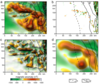Figure 3

The intensity of the magnetic anomalies reaches 1000–1500 nT (Figure 3a). Digital data from the map of the Ural region were used to study the structure of the anomalous magnetic field [Chursin et al., 2008]. The anomalies from magnetized massifs in the upper layer of the Earth's crust to the depth of 5 km were identified using up-down transformations for the conversion height $H=5$ km and were obtained by subtracting these data from the initial field (Figure 3b). For magnetized sources located in deeper layers below 5 km, up-down calculations were performed for $H=20$ km. Anomalies from magnetized blocks in the granite layer were obtained as the difference between the calculated fields for $H=5$ and 20 km (Figure 3c). The magnetic field calculated for $H=20$ km consists of long-wave regional anomalies that correspond to the integral distribution of magnetization in the lower basalt layer of the Earth's crust (Figure 3d).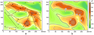Figure 4

For the separated magnetic anomalies of the module induction $\Delta T$, approximation was performed by singular sources – rods magnetized along the modern geomagnetic field. For the regional component of the magnetic field, the approximation results and the segment's locations, as well as their depths are shown in Figure 4a. Under the epicenter zones of the anomalies, the depths to the sources are minimal and amount to 16 km. Then, the anomalous fields were reduced to the magnetic pole and, using the direct problem, the values of the vertical component $Z$ were calculated for the found parameters of the sources with vertical magnetization. The difference between the anomalies of the module induction $\Delta T$ and $Z$ is shown in Figure 4b. Despite the fact that our region is located at high latitudes of $56\mbox{°}-58\mbox{°}$ and the geomagnetic field vector has an inclination close to vertical $73\mbox{°}$, the epicenters of the $Z$ anomalies are shifted 12–15 km north of the epicenters of the total induction anomalies (Figure 4b).

To solve the inverse problem, the magnetic field data from each layer were specified on grids of $100 \times 100$ points. As a result of an iterative process, the geometry of the sources in each layer was determined, while the relative deviation error of the initial and model anomalies was less than 1–3 percent, with the exception of the edges of the region of 10–20 km. Therefore, we reduced the region and cut our models 20 km from each edge. When choosing the source magnetization in the layers, we based on the results of previously performed calculations along seismic profiles, as well as three-dimensional models of regional anomalies [Fedorova, 2005; Fedorova and Rublev, 2019; Fedorova et al., 2017]. The main carriers of magnetization in the Earth's crust are the minerals of the titanomagnetite series and, above all, magnetite. As a rule, these minerals are found in significant quantities only in basic and ultramafic rocks, which can have a high magnetization of 2–6 A/m. Sedimentary rocks contain a small amount of magnetic minerals and this layer does not create noticeable magnetic anomalies. The average magnetization of the granite layer is low, not exceeding 0.3 A/m. According to geothermal estimates, in our region, at depths of more than 30–35 km, the temperature exceeds $580\mbox{°}$ (Curie point of magnetite) and the minerals lose their ferromagnetic magnetization. Therefore, it is possible to limit the magnetic sources in a basalt layer 30 km deep. Models of sources of the magnetic anomalies in the granite and basalt layers were built for magnetization of 3 and 4 A/m.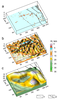Figure 5

The modeling results in the form of a three-dimensional surface of the sources in the layers are shown in Figure 5.

In the upper layer (Figure 5a), small sources are present within the East European platform but their depth is more than 4.5 km. In the Urals, east of the Main Uralian Fault, magnetized massifs are located close to the surface of the Earth, which is in good agreement with the data of geological mapping. To the west the Tagilo-Magnitogorskian megazone are bordered by serpentinitic melange. This megazone predominantly consists of Ordovician–Lower Carboniferous complexes of oceanic crust and island arc, including the Platinum-bearing Belt of layered basic-ultramafic massifs, overlain by platformal carbonate and rift-related volcanic rocks [Puchkov, 2009].

In the granite layer, magnetized sources form linear belts 100–200 km long (Figure 5b). Probably, on the East European platform, these are green belts formed at different Precambrian times. The strike of the zones varies from sub-latitudinal to submeridional, which indicates a long and complex history of the formation of the ancient platform. In the Urals, 3 belts are extended in the north-south direction. They correspond to the deep-Uralian faults, which extend along the Ural structures for more than 3000 km.

The basalt layer has 5 large elevations in the form of domes, which rise up to 15–17 km, and the surface deflections reach 26 km. (Figure 5c). Note that the basaltic layer domes are present not only within the East European platform, but also under the Urals structures up to the Main Uralian Fault. Consequently, the upper layer of 15–20 km of the Earth's crust of the Urals within the Western and Central megazones was formed as a result of thrust from the east and lies on the ancient basaltic layer of the Precambrian platform.

We note that values of the regularization parameter in equation (3) depend on downward depth. For example, if depth is 5 km then $\alpha= 0.23$, if depth is 20 km then $\alpha= 0.208$.

### 4. Conclusion

With a help of the new computer technology, the structural features of the anomalous magnetic field for the Western Urals territory were studied. Anomalies from various layers of the Earth's crust were identified and the models of the sources of magnetic anomalies in the granite layer and the surface of the basaltic layer were constructed. The study revealed basic-ultramafic massifs under a thick sedimentary cover, as well as extended deep belts and large elevations of the basalt layer of the Earth's crust. The results clarify the lithosphere structure of the Western Urals and can be used for geodynamic reconstructions.

#### Acknowledgments

The Russian Science Foundation (Grant No. 20-17-00058) is acknowledged for supporting this research.

### References

Byzov, D., L. Muravyev, N. Fedorova (2017) , The approximation of anomalous magnetic field by array of magnetized rods, AIP Conference Proceedings, 1863, p. 560051, https://doi.org/10.1063/1.4992734.

Chursin, A. V., A. M. Prut'yan, N. V. Fedorova (2008) , Digital anomalous magnetic field map of the Northern, Middle, Southern Urals and adjacent territories of the East-European and West-Siberian platforms, Litosfera, 6, p. 63–72 (in Russian).

Druzhinin, V. S., P. S. Martyshko, et al. (2015) , Tectonodynamic Model of the Earth's Crust of the Urals and Neighboring Areas, Doklady Earth Sciences, 463, no. 1, p. 659–662, https://doi.org/10.1134/S1028334X15070090.

Fedorova, N. V. (2005) , Modeling of the magnetic field variation in relation to the origin of the Manchazh secular trend anomaly, Izvestiya. Physics of the Solid Earth, 41, no. 5, p. 355–362.

Fedorova, N. V., A. L. Rublev (2019) , Numerical Modeling of Magnetic Anomalies Sources in the South Urals Earth Crust, Russian Geology and Geophysics, 60, no. 11, p. 1310–1318, https://doi.org/10.15372/RGG2019106.

Fedorova, N. V., A. L. Rublev, et al. (2017) , Magnetic anomalies and model of the magnetization in the Earth's crust of circumpolar and polar the sectors of Ural region, Geofizicheskiy Zhurnal, 39, no. 1, p. 111–122, https://doi.org/10.24028/gzh.0203-3100.v39i1.2017.94014.

IAGA, (1995) , Division V, Working Group 8, International Geomagnetic Reference Field, 1995 Revision, J. Geomag. Geoelectr., 47, no. 12, p. 1257–1261, https://doi.org/10.5636/jgg.47.1257.

Lavrentiev, M. M. (1967) , Some Improperly Posed Problems of Mathematical Physics, 76 pp., Springer, New York, https://doi.org/10.1007/978-3-642-88210-4.

Martyshko, P. S., I. L. Prutkin (2003) , Technology for separating the gravity sources by the depth, Geofiz. Zh., 25, no. 3, p. 159–170, https://doi.org/10.3997/2214-4609-pdb.38.F193 (in Russian).

Martyshko, P. S., N. V. Fedorova, et al. (2014) , Studying the Structural Features of the Lithospheric Magnetic and Gravity Fields with the Use of Parallel Algorithms, Izvestiya. Physics of the Solid Earth, 50, no. 4, p. 508–513, https://doi.org/10.1134/S1069351314040090.

Martyshko, P. S., I. V. Ladovskii, A. G. Tsidaev (2010) , Construction of regional geophysical models based on the joint interpretation of gravitaty and seismic data, Izvestiya. Physics of the Solid Earth, 46, no. 11, p. 931–942, https://doi.org/10.1134/S1069351310110030.

Mayer, V. I., F. I. Nikonova, N. V. Fedorova (1985) , A numerical optimization procedure for interpreting gravity and magnetic-anomalies, Izvestiya, Fizika Zemli, 5, p. 46–57.

Olsen, N. (2002) , A model of geomagnetic field and its secular variation for epoch 2000 estimated from Orsted data, Geophysical Journal International, 149, no. 2, p. 454–462, https://doi.org/10.1046/j.1365-246X.2002.01657.x.

Prutkin, I. L. (1986) , The solution of three-dimensional inverse gravimetric problem in the class of contact surfaces by the method of local corrections, Physics of the Solid Earth, 22, no. 1, p. 49–55.

Puchkov, V. N. (2009) , The evolution of the Uralian orogen, Geological Society, London, Special Publications, 327, no. 1, p. 161–195, https://doi.org/10.1144/SP327.9.

Received 22 March 2021; accepted 20 April 2021; published 21 May 2021.Citation: Martyshko P. S., N. V. Fedorova, A. L. Rublev (2021), Numerical algorithms for structural magnetometry inverse problem solving, Russ. J. Earth Sci., 21, ES3002, doi:10.2205/2021ES000766.

Generated from LaTeX source by ELXfinal, v.2.0 software package.